# Number Bonds to 10

Number Bonds to 10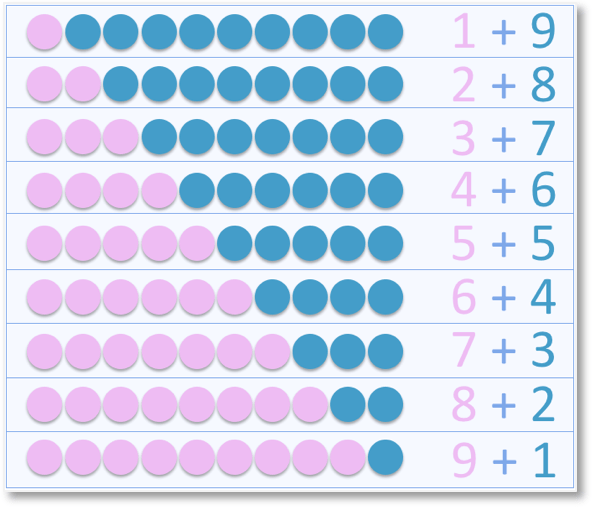• Number bonds to 10 are pairs of numbers that add to make ten.
• The number bonds to ten are:
• 1 + 9.
• 2 + 8.
• 3 + 7.
• 4 + 6
• and 5 + 5• Number bonds are important for providing a visual understanding of the size of a number.
• Number bonds help with understanding addition and subtraction.
• Number bonds help to show that there are many ways in which one number can be formed or broken down.
• When teaching number bonds to 10, remember that there are only 5 separate pairs of numbers to learn.
• Remembering one number bond pair can help to learn the rest.
• For example, starting with 5 + 5, we can increase one number and decrease the other to form 6 + 4.

Number Bonds to 10 are pairs of numbers that add to make 10.

There are 5 pairs of numbers to learn.#### Number Bonds to 10: Interactive Activity

Number Bonds to 10: Interactive Questions

# What are Number Bonds to 10?

Number bonds to 10 are pairs of numbers that add together to equal 10.

The poster below shows the complete list of number bonds to 10.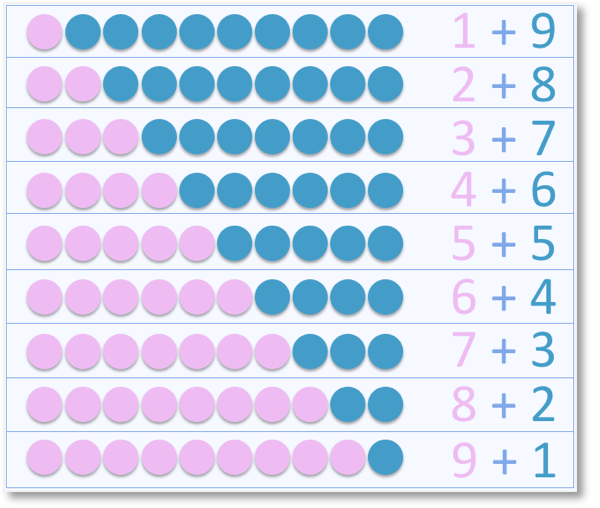Number bonds to 10 are usually one of the first set of number facts that are taught.

Number Bonds to 10 are usually taught to primary school children in their first or second year (KS1 or First Grade).

Number bonds are important for developing further skills such as addition and subtraction. Learning number bonds to 10 then lead on to learning number bonds to 20 and further number facts up to 100. Number bonds also help children to visualise the size of numbers.

It is important to teach number bonds to 10 visually, with practical activities when first introducing them. This can be done with counters as shown below.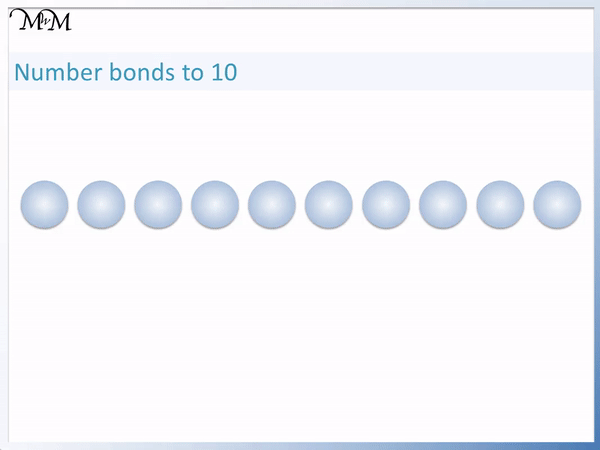Tens frames can be used as a guide to put the counters in. Tens frames are simple blank rectangles that are 2 by 5 in size.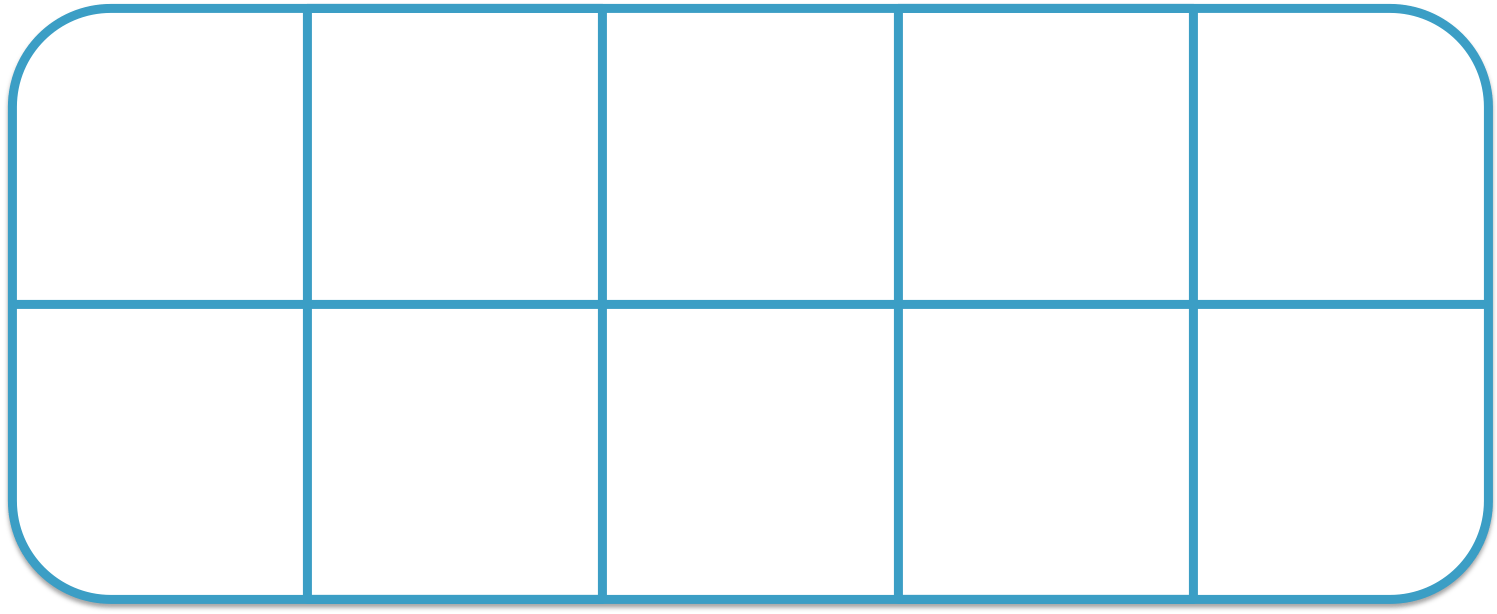To use a tens frame, simply shade in some of the boxes (or put counters in) and then see how many more filled boxes are required to fill the complete frame of 10.

For example, here is a tens frame already containing 2 counters.

It is used to work out which number bond pairs with 2 to make 10.

To use a tens frame, add a counter to each blank box and then count how many counters have been added.8 more counters are needed to fill the frame.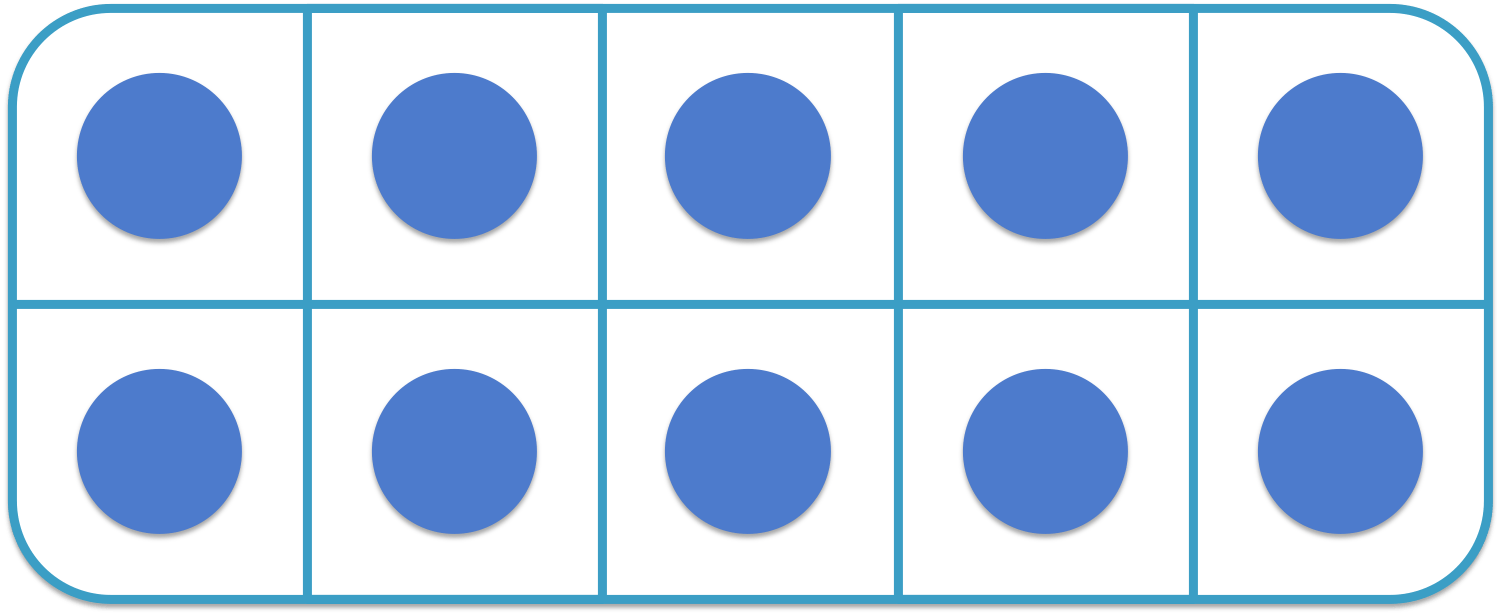When introducing the idea of number bonds, further practical activities can be used, such as multi-link cubes or dominoes.

It can help to give time to practise with physical models in order to develop a good understanding of how the number 10 can be broken down into different sums.

However to memorise number bonds to 10, we can see that there are only five pairs of numbers to remember.The pairs of numbers that add to make 10 are:

• 1 and 9
• 2 and 8
• 3 and 7
• 4 and 6
• 5 and 5Remembering 5 pairs of numbers is easier than remembering 10 separate addition facts.

For example: 1 + 9 contains the same pair of numbers as the number bond of 9 + 1.

We simply swap the positions of the two numbers.

When teaching number bonds to 10, it is useful to break it down into the task of remembering the 5 pairs of numbers that add to make 10.

If you remember one of the number bonds to 10, then you can use this to work out some of the others.

The pairs of numbers that add to make 10 are shown again below and we can see a pattern with the numbers.

As the first number increases from 1 to 5, the second number decreases from 9 to 5.

• 1 and 9
• 2 and 8
• 3 and 7
• 4 and 6
• 5 and 5

We can get to another number bond by adding 1 to one of the numbers and subtracting one from the other number.

For example if we remember 5 + 5 , then we can add 1 to one number and subtract 1 from the other to make 6 + 4.

If we remember 1 + 9, we can add 1 to the first number and subtract 1 from the second number to make 2 + 8.

In order to move swiftly on to learning the number bonds to 20 and further addition facts, it is important to know the number bonds to 10 with quick recall.

Part, part whole models are often used for practising number bonds to 10.

Part, part whole models contain 3 circles with a larger circle connected to two smaller circles.

The larger circle contains the number bond we are making, which in this case is 10.

One of the smaller circles contains one part of the number bond and the other smaller circle is blank, ready to be filled in.We have part part whole model worksheets for download with which you can practise this.

Here are the complete set of number bonds to 10 shown with the part part whole model.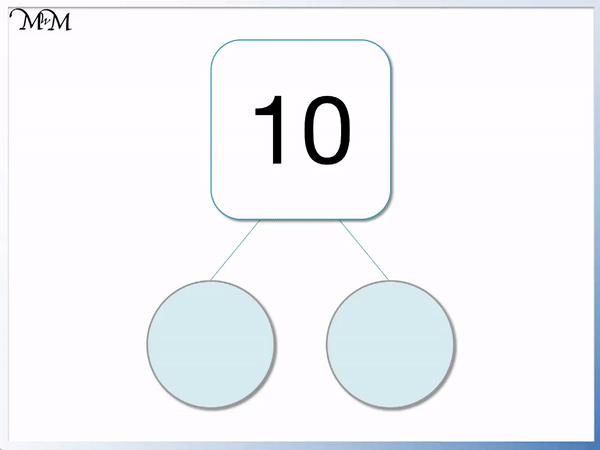Games, competitions and quick tests are very useful ways to practise and we have Interactive Questions that you can use.Now try our lesson on Number Bonds to 20 where we learn the pairs of numbers that add to make 20.